## Monday, November 22, 2010

### Irodov Problem 3.64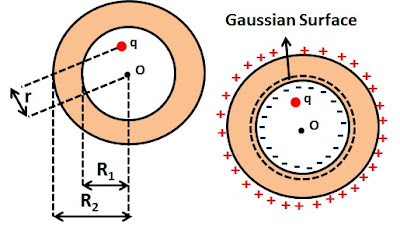First let us start by understanding some important concepts. As described in Problem 3.63, the entire conductor must have the same potential and hence, there cannot be any electric field inside the conductor. In other words there is no electric field within the conducting shell.

Consider a Gaussian spherical surface of radius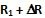such that .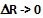, in other words this Gaussian surface is just above the inner circle within the conductor. Since there cannot is any electric field in side the conductor, there is no electric flux through the Gaussian surface. From Gauss Law in turn, this imples that the net charge contained within this Gaussian sphere must be 0. This can only happen if there is a net charge of -q on inner the surface of the sphere. Let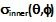be the charge density at a location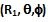in spherical coordinates on the inner surface of the conductor. Then we have,Since the electric field anywhere within the conductor is 0, for all Gaussian surfaces in the conducting shell with radius R such thatthe net flux through them will be zero, in turn implying that no free charges now exist anywhere within the shell. Since the total charge in the conductor must be consrved, this in turn implies that all the positive charges in the shell are concentrated on the outer surface of the conducting shell as shown in the figure. Further, the sum total of all these positive charges will be equal to q. Letbe the surface charge density on the outer surface of the conductor at a location with sperical coordinates. Then, we have,The potential at the center of the sphere is due to three sources, i) the charge q, ii) the surface charge density at the inner surface of the conducting sphere and iii) the surface charge density at the outer surface of the conducting sphere and is given by.#### 1 comment:

1.This comment has been removed by the author.